# Existence of directional derivatives in every direction not implies differentiable

## Statement

### For a function of two variables at a point

It is possible to have a function$f$ of two variables$x,y$ and a point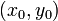$(x_0,y_0)$ in the domain of$f$ such that$f$ has a directional derivative in every direction at$(x_0,y_0)$, but$f$ is not differentiable at$(x_0,y_0)$, i.e., the gradient vector of$f$ at$(x_0,y_0)$ does not exist.

### For a function of two variables overall

It is possible to have a function$f$ of two variables$x,y$ and a point$(x_0,y_0)$ in the domain of$f$ such that$f$ has a directional derivative along every direction at every point but$f$ is not differentiable everywhere, i.e., there exists a point where the gradient vector of$f$ does not exist.

### For a function of multiple variables

We can replace functions of two variables by functions of more than two variables.

## Proof

### Example

Consider the function: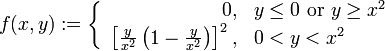$f(x,y) := \left\lbrace \begin{array}{rl} 0, & y \le 0 \mbox{ or } y \ge x^2 \\ \left[\frac{y}{x^2}\left(1 - \frac{y}{x^2}\right)\right]^2, & 0 < y < x^2 \\\end{array}\right.$

Alternatively, we can describe it as: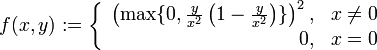$f(x,y) := \left\lbrace \begin{array}{rl} \left(\max \{ 0, \frac{y}{x^2}\left(1 - \frac{y}{x^2}\right) \}\right)^2, & x \ne 0 \\ 0, & x = 0 \\\end{array}\right.$

We first argue that$f$ is continuous at all points other than$(0,0)$:

• At any point with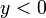$y < 0$, the function is the zero function around the point, hence is differentiable with gradient vector the zero vector.
• At any point with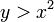$y > x^2$, the function is the zero function around the point, hence is differentiable with gradient vector the zero vector.
• At any point with$0 < y < x^2$, the function has the rational function description$\left(\frac{y}{x^2}\left(1 - \frac{y}{x^2}\right)\right)^2$ around the point, hence is differentiable with gradient vector the zero vector.
• At any point on the line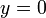$y = 0$ other than the origin, there are two definitions of the function around the point: the definition 0 (from the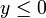$y \le 0$ side), and the definition$\left(\frac{y}{x^2}\left(1 - \frac{y}{x^2}\right)\right)^2$ (from the$0 < y < x^2$ side). Both definitions evaluate to zero at the point, and the gradient vectors corresponding to both definitions are zero vectors, so the function is differentiable and its gradient vector is the zero vector.
• At any point on the curve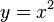$y = x^2$ other than the origin, there are two definitions of the function around the point: the definition 0 (from the$y \ge x^2$ side), and the definition$\left(\frac{y}{x^2}\left(1 - \frac{y}{x^2}\right)\right)^2$ (from the$0 < y < x^2$ side). Both definitions evaluate to zero at the point, and the gradient vectors corresponding to both definitions are zero vectors, so the function is differentiable and its gradient vector is the zero vector.

We now argue that$f$ has directional derivative zero in every linear direction at$(0,0)$. It suffices to consider half-line directions because continuity from a linear direction is continuity from both half-line directions.

• For the half-line directions that are below or along the$y = 0$ line, the function is identically zero along the half-line, so the directional derivative is zero.
• For the half-line directions that are above$y = 0$, we note that, sufficiently close to the origin, this half-line is completely above the$y = x^2$ curve (explicitly, if the slope of the line is$m$, then the half-line is above$y = x^2$ for$|x| < |m|$). Thus, sufficiently close to the origin,$f$ looks like the zero function on this half-line. Thus, the directional derivative is zero.

We finally demonstrate that$f$ is not continuous at$(0,0)$ by finding a curve approaching the origin along which the limit at the origin is not zero. Consider the curve:$y = \frac{1}{2}x^2$

Consider the limit:$\lim_{x \to 0^+} f(x,y) = \lim_{x \to 0^+} f(x,x^2/2) = \lim_{x \to 0^+} (1/2)(1 - 1/2) = \lim_{x \to 0^+} 1/4 = 1/4 \ne 0$

Since the function is not continuous at$(0,0)$, it cannot be differentiable and cannot have a gradient vector at$(0,0)$.